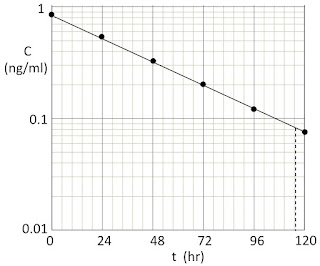## Friday, October 23, 2015

### Clearance and Semilog Plots

I occasionally like to write a new homework problem as a gift to the readers of Intermediate Physics for Medicine and Biology. Here is the latest, for Section 2.5 about clearance.
Problem 11 ½. A patient has been taking the drug digoxin for her atrial fibrillation. Her distribution volume is V = 400 l. At time t = 0 she stops taking the drug and her doctor measures her blood digoxin concentration, C, every 24 hours.

 t (hr) C (ng/ml) 0 0.85 24 0.53 48 0.33 72 0.20 96 0.125 120 0.077

Calculate the clearance, K, in ml/min.
I like this problem because it reinforces two concepts at once: 1) clearance, and 2) using semilog plots. First, let’s analyze clearance. Equation 2.21 in IPMB gives the blood concentration as a function of time

C(t) = Co exp(−(K/V)t) .

If we can measure the rate of decay of the concentration, b, where the exponential factor is written as exp(−bt), we can calculate the clearance from K = bV. So, this problem really is about estimating b from the given data.

To calculate the rate b, we should plot the data on semilog graph paper. You can download this graph paper (for free!) at http://www.printablepaper.net/category/log, http://customgraph.com, or http://www.intmath.com/downloads/graph-paper.php. The figure below shows the data points (dots).The concentration as a function of time.

Now, draw a line through the dots. Sophisticated mathematical techniques could be used to fit the best line through this data, but for this homework problem I suggest merely fitting a line by eye. For data with no noise, such as used here, you should be able to calculate b to within a few percent using a ruler, pen, and some care.

Next, our goal is to determine the decay constant b from the equation C = Co exp(−bt) using the method discussed in Section 2.3. Select two points on the line. They could be any two, but I suggest two widely spaced points. I’ll use the initial data point (t = 0, C = 0.85) and then estimate the time when the line has fallen by a factor of ten (C = 0.085). The vertical dashed line in the above figure indicates this time, which I estimate to be t = 115 ± 2 hr. I include the uncertainty, which reflects my opinion that I can estimate the time when the dashed line hits the time axis to slightly better than plus or minus one half of one of the small divisions shown on the paper, each of which is 24/5 = 4.8 hr wide. So I have two equations: 0.85 = Co and 0.085 = Co exp(−115b). When I divide the two equations, Co cancels out and I find 10 = exp(115b). Therefore, b = ln(10)/115 = 0.0200 ± 0.0003 hr−1. If I write the exponential as exp(−t/τ), then the time constant is τ = 1/b = 50.0 ± 0.9 hr. Often we say instead that the half-life is t1/2 = ln(2) τ = 34.7 ± 0.6 hr.

At this point, I suggest you inspect the plot and see if your result makes sense. Does the line appear to drop by half in one half-life? Using the plot, at t = 35 hr I estimate that C is about 0.42, which is just about half of 0.85, so it looks like I’m pretty close.

Now we can get the clearance from K = bV = (0.02 hr−1)(400 l) = 8 l/hr. The problem asks for units of ml/min (a common unit used in the medical literature), so (8 l/hr)(1 hr/60 min)(1000 ml/l) = 133 ml/min. The uncertainty in the clearance is probably determined by the uncertainty in the distribution volume, which we are not given but I’d guess is known to an accuracy of no better than 10%.

Astute readers might be thinking “This is a great homework problem, but I don’t understand why the distribution volume is so big; 400 l is much more than the volume of a person!” The distribution volume does not represent an actual volume of blood or of body fluid. Rather, it takes into account that most of the digoxin is stored in the tissue, with relatively little circulating in the blood. But since the blood concentration is what we measure, the distribution volume must be “inflated” to account for all the drug stored in muscle and other tissues. This is a common trick in pharmacokinetics.

I believe that analyzing data with semilog or log-log graph paper is one of those crucial skills that must be mastered by all science students. As an instructor, you cannot stress it enough. Hopefully this homework problem, and ones like it that you can invent, will reinforce this technique.